In :
import pandas as pd
import matplotlib.pyplot as plt
import seaborn as sns
import numpy as np


# Feature Selection with Lasso, Ridge, ElasticNet in Python and "scikit-learn"¶

Today we'll use the "scikit-learn" (aka sklearn) implementation of these models. "scikit-learn" is a machine learning-focused library that has a ton of helper functions for data scientists to quickly and efficiently try a lot of different models.

## Prerequisites: Statquest Playlist¶

Since this notebook is mainly to show you how to implement these models in python, you should watch this Statquest playlist to get the intuition behind these models:

## The sklearn workflow¶

The most basic sklearn model workflow goes something like this:

Step Action Example
0 Import the model from sklearn from sklearn.linear_model import LinearRegression
1 Instantiate the model class model = LinearRegression()
2 Fit the model model.fit(X, y)
3 Use the fitted model to make a prediction model.predict(X)

This standard interface is what makes sklearn so powerful - with .fit() and .predict() and a huge variety of models in the library, you can use this common language to try all sorts of machine learning models.

And that's just the start, from this basic 3-step pipeline you can add all kinds of additional steps to this worfklow that make experimentation very convenient for the user.

## Example: Linear Regression in sklearn¶

Before we cover Lasso, Ridge, and ElasticNet in sklearn it might be helpful to demonstrate some basic functionality with doing a linear regression.

Reference: Linear Regression

In :
# Import the model
from sklearn.linear_model import LinearRegression


Next we'll load in our data. We'll be using seaborn, a visualization library that has some 'toy' datasets to play with and we'll also use it briefly for some specialized plots.

The mpg dataset is a famous dataset that has some car engine attributes like engine displacement (engine size) and others that can be used to estimate the car's fuel efficiency, measured in miles per gallon or mpg.

We can load it in using the sns.load_dataset() function:

In :
# load mpg example dataset
# we'll filter on just a few columns and drop na/empty values
mpg = mpg[['mpg', 'displacement', 'horsepower', 'weight']].dropna()
X = mpg[['displacement', 'horsepower', 'weight']]
y = mpg['mpg']


And one helpful plot for you would be the sns.pairplot function that will plot scatterplots for each of the variables against each other and also display the distribution for each variable as a histogram:

In :
# Convenience method to plot pairs of variables together all in one go!
sns.pairplot(mpg)

Out:
<seaborn.axisgrid.PairGrid at 0x7ff5f0b498e0>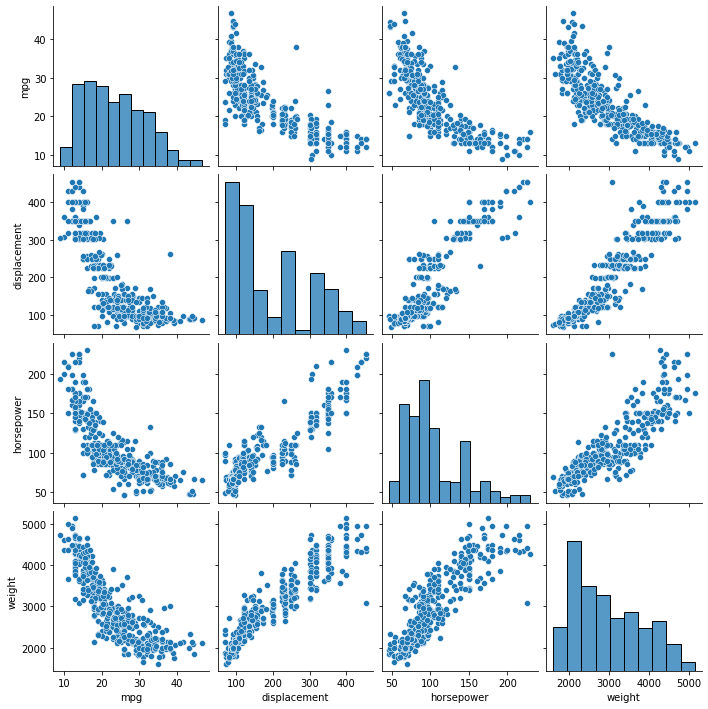Next, we'll follow the sklearn workflow.

Let's model the relationship between average MPG and engine displacement and get a quick $R^2$ value:

In :
# Filter on just the 'displacement' variable in the X dataframe
x = X[["displacement"]]

model = LinearRegression()
model.fit(x, y)

# model.score() returns the model R^2 in this case
R2 = model.score(x, y)
print("Model R^2: ", R2)

Model R^2:  0.6482294003193044

In :
# Show the fitted values
yhat = model.predict(x)

plt.scatter(x, y, label="Engine Displacement")
plt.plot(x, model.predict(x), label="Fitted model", c="r")
plt.title("MPG ~ Displacement")
plt.legend()
plt.show()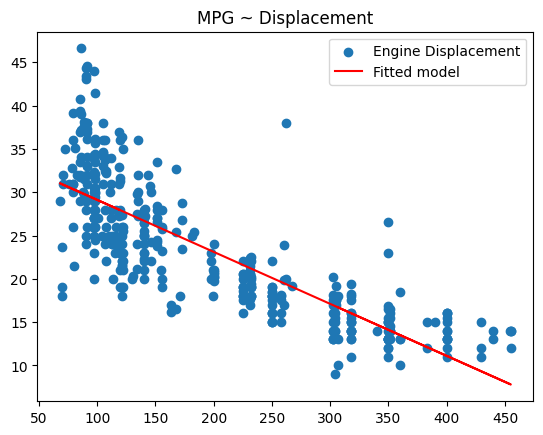With Linear Regression and similar models, we can extract the fitted coefficients and the intercept with two commands:

1. model.coef_ will return the coefficients
2. model.intercept_ will return the intercept
In :
print("model coefficient(s):", model.coef_)
print("model intercept:", model.intercept_)

model coefficient(s): [-0.06005143]
model intercept: 35.12063593840391


Notice that this has fewer "batteries included" than statsmodels but it gets the job done. So if you wanted other summary statistics like p-values you will need to do them manually in sklearn.

But all that aside, in math terms, we've just fit the model:

$$\hat{Y} = -0.06x + 35.12$$

where $x$ is the engine displacement and $\hat{Y}$ is the estimated average value of mpg. In other words, for every unit increase in engine displacement, average mpg is expected to decrease by -0.06.

# Feature Selection with Lasso/Ridge/ElasticNet¶

In :
from sklearn.linear_model import Lasso, Ridge, ElasticNet

# For this exercise we'll split the data into training and test sets
from sklearn.model_selection import train_test_split
# And we'll need to scale the data
from sklearn.preprocessing import StandardScaler


sklearn Reference:

## Scaling your data¶

With regularization models you must scale your data!

Since we're dealing with penalties, we need to standardize the scale of data so the regularization penalty doesn't over-penalize numbers that are orders of magnitude higher than others.

The StandardScaler() class in sklearn helps us with this, and using it is simple:

1. Instantiate the scaler class with StandardScaler()
2. fit the scaler with the .fit() method on the data
3. Finally, transform the data with .transform()
1. Alternatively, you can combine both the .fit and .transform steps with a single .fit_transform() call

And this particular scaler simply takes the X data and standardizes them into Z-scores by subtracting the mean and dividing over the standard deviation, i.e.:

$$\text{scaled horsepower} = \frac{\text{horsepower} - \text{average horsepower}}{\text{horsepower standard deviation}}$$
In :
# Here's the data again for clarity
X = mpg[['displacement', 'horsepower', 'weight']]
y = mpg['mpg']

# Instantiate the Scaler class
scaler = StandardScaler()
# Fit and then transform the X-data
scaled_X = scaler.fit_transform(X)
# The transformed data is now an array, we want to convert it back
# to a dataframe for easier parsing (optional)
scaled_X = pd.DataFrame(scaled_X, columns=['displacement', 'horsepower', 'weight'])


note: This transformation is a little messy because I'm scaling only the X values (i.e. the engine displacement, horsepower, and weight) and leaving the y unscaled to help a little with the interpretation piece. This code is normally much simpler when you are scaling the y values as well.

In :
# We'll take our scaled X values and combine it with the unscaled y
df = scaled_X.copy()
df['mpg'] = y
df = df.dropna()


### train_test_split()¶

The next step we will perform is to divide the data into two parts: a training set and a test set.

In :
# split the new dataframe into train/test
mpg_train, mpg_test = train_test_split(df, test_size=0.20, random_state=42)

X_train = mpg_train[['displacement', 'horsepower', 'weight']]
X_test = mpg_test[['displacement', 'horsepower', 'weight']]
y_train = mpg_train['mpg']
y_test = mpg_test['mpg']


## Lasso Regression¶

Statquest video: Regularization Part 2: Lasso (L1) Regression

First model we'll demonstrate is a single call of Lasso and manually set the value of the alpha parameter.

Lasso Regression) penalizes the less important features by reducing their coefficients (possibly to zero), unlike Ridge Regression which merely shrinks the coefficients for the less important features but cannot set them to zero entirely.

In :
model = Lasso(alpha=0.01, random_state=42)
model.fit(X_train, y_train)
R_2 = model.score(X_test, y_test)

# print the score rounded to two digits
print("R^2 Value: ", np.round(R_2,2))

R^2 Value:  0.27


So our simple Lasso model fit on the training data resulted in a $R^2$ of 0.27 on the test data. That is not so good! But the important piece is that we should look at the model coefficients:

In :
print("intercept: ", model.intercept_)
pd.DataFrame({"feature": model.feature_names_in_,
"coefficients":model.coef_})

intercept:  23.348832405776655

Out:
feature coefficients
0 displacement -2.317209
1 horsepower -1.852412
2 weight -0.818235

### How do I interpret this?¶

So, in math terms, here's what the above model is saying:

$$\text{expected mpg} = -2.32(\text{displacement}) - 1.85(\text{horsepower}) - 0.82(\text{weight}) + 23.35$$

So for every unit increase in displacement we expect to see a decrease in mpg by 2.32 miles per gallon. That's a lot! But note here that we scaled the displacement, horsepower, and weight numbers. If we want to convert these coefficients back to the original scale, we'll need to do a little math. I'll skip it for now but just know that it's possible in this situation.

But this is a post on feature importance, so how would you do this with Lasso, Ridge, or ElasticNet?

### Feature Importance with Lasso¶

So we notice that with the alpha parameter at a low value of 0.01, Lasso didn't send any of these variables to zero. If we were to increase the alpha parameter, we would see that some of these coefficients would go to zero.

So one way of interpreting feature importance with Lasso or Ridge is to try different values of alpha and see what happens to the coefficients.

The code below shows an example:

In :
coefs = []
alphas = np.arange(0.01,10, 0.01)
for a in alphas:
model = Lasso(alpha=a, random_state=42)
model.fit(X_train, y_train)
coefs.append(model.coef_)


Then we'll do a plot of the coeffient values vs alpha.

In :
ax = plt.gca()

ax.plot(alphas, coefs, label=['displacement', 'horsepower', 'weight'])
ax.set_xscale("log")
ax.set_xlim(ax.get_xlim()[::-1])  # reverse axis
plt.xlabel("log(alpha)")
plt.ylabel("coefficients")
plt.title("Lasso coefficients as a function of regularization strength")
plt.axis("tight")
plt.legend()
plt.show()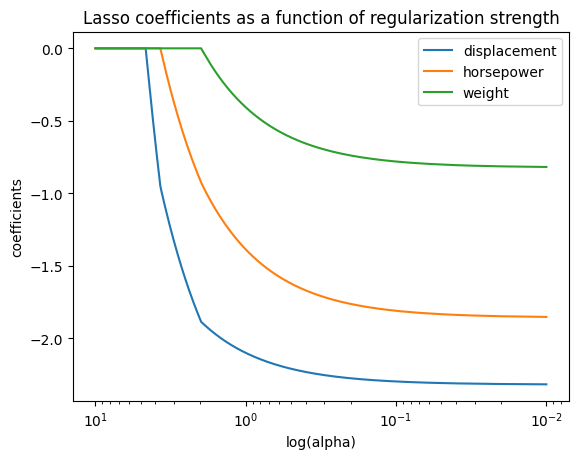### How do you read this?¶

So you might notice a few funky things about this plot. I reversed the x-axis so that alpha decreases from left to right, so regularization strength is decreasing from left to right. And the coefficients are negative before going to zero with higher values of alpha. A little confusing but it's a convention.

But notice how we have an order in which the lines go to zero. In the above example, weight is the first value to go to zero as alpha increases, followed by horsepower, and then displacement. This gives you a relative idea of the feature importance which might be helpful in your understanding of the problem.

For another example with Ridge Regression, check this out

Note: Another term for this kind of plot is a 'path' plot, and sklearn has some helper functions, see example

### How do you find the best value of alpha for prediction accuracy?¶

Now, another twist on the problem is if your goal isn't learning feature importance and your goal is to do prediction and let the models do the shrinkage for you, then you can repeatedly try a lot of different values of alpha and find the value that gives you the least error on the test set. In the below example we'll use root mean squared error (RMSE) as our error metric that we're optimizing for:

In :
yhat = model.predict(X_test)
RMSE = (np.mean((yhat - y_test)**2))**0.5
print(RMSE)

7.019453139121642


Then we iterate over several values of alpha:

In :
errors = []
alphas = np.arange(0.01,10, 0.01)
for a in alphas:
model = Lasso(alpha=a, random_state=42)
model.fit(X_train, y_train)
yhat = model.predict(X_test)
RMSE = (np.mean((yhat - y_test)**2))**0.5
errors.append(RMSE)

In :
ax = plt.gca()

plt.scatter(alphas, errors)
ax.set_xscale("log")
plt.xlabel("log(alpha)")
plt.ylabel("RMSE")
plt.title("Lasso Test RMSE at various values of alpha")
plt.show()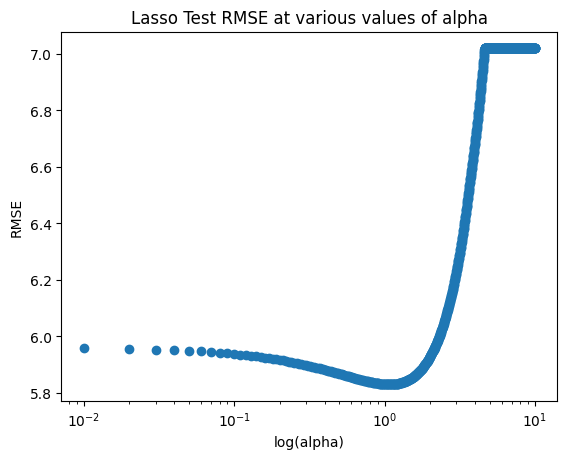In :
best_alpha = alphas[np.argmin(errors)]
print("best_alpha = ", best_alpha)

best_alpha =  1.07


We can then plug that best alpha back in to our model:

In :
model = Lasso(alpha=best_alpha, random_state=42)
model.fit(X_train, y_train)
R_2 = model.score(X_test, y_test)

# print the score rounded to two digits
print("Test R^2 Value: ", np.round(R_2,2))

Test R^2 Value:  0.3


So, we get a minor improvement!

In :
pd.DataFrame({"feature": model.feature_names_in_,
"coefficients":model.coef_})

Out:
feature coefficients
0 displacement -2.084907
1 horsepower -1.353816
2 weight -0.377263

And notice here that since we were optimizing for RMSE on the test set, we found a value of alpha that shrunk the coefficients but didn't set any of them to zero. If we had increased alpha more we would eventually see weight fall off.

#### Visualizing the model fit¶

So since we are dealing with multivariable regression we can't make a 4-D plot to show the 4-D hyperplane that this model fit. But we can kind of get a sense by plotting just a single predictor variable (i.e. 'horsepower') against the value of mpg to get some intuition:

In :
y_fitted = model.predict(X_train)
plt.scatter(X_train[["horsepower"]], y_train, label="Training Data")
plt.scatter(X_train[["horsepower"]], y_fitted, label="Fitted Values", c="r")
plt.title("Lasso ")
plt.xlabel("scaled horsepower")
plt.ylabel("mpg")
plt.legend()
plt.show()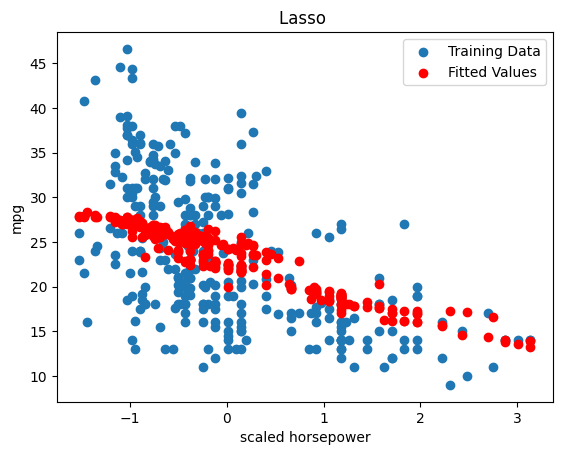But, can we do better?

## How do you find alpha?¶

In practice you could iteratively try many many different kinds of alpha until you arrived at the best answer - and you could do this manually (as we just did above) but sklearn has helper functions that already do this for you.

Here we'll use 5-fold cross validation to find the ideal value of alpha from the training set, and then use that fitted model on the test set.

sklearn has LassoCV, RidgeCV, and ElasticNetCV implementations for our convenience. Here we'll repeat the analysis from above as a demo with these three.

In :
from sklearn.linear_model import LassoCV, RidgeCV, ElasticNetCV

In :
model = LassoCV(cv=5, random_state=0).fit(X_train, y_train)
print(model.score(X_test,y_test))

y_fitted = model.predict(X_train)
plt.scatter(X_train[["horsepower"]], y_train, label="X")
plt.scatter(X_train[["horsepower"]], y_fitted, label="Fitted", c="r")
plt.legend()
plt.show()

0.26601042348436355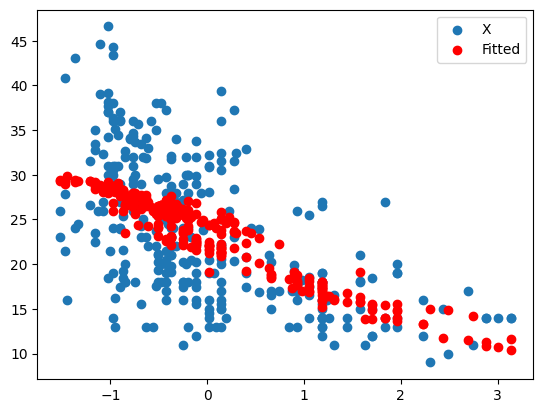In :
model.alpha_

Out:
0.004619906312005148
In :
pd.DataFrame({"feature": model.feature_names_in_,
"coefficients":model.coef_})

Out:
feature coefficients
0 displacement -2.318341
1 horsepower -1.854957
2 weight -0.820504
In :
model = RidgeCV(cv=5).fit(X_train, y_train)
print(model.score(X_test, y_test))

y_fitted = model.predict(X_train)
plt.scatter(X_train[["horsepower"]], y_train, label="X")
plt.scatter(X_train[["horsepower"]], y_fitted, label="Fitted", c="r")
plt.legend()
plt.show()

0.2709055015774047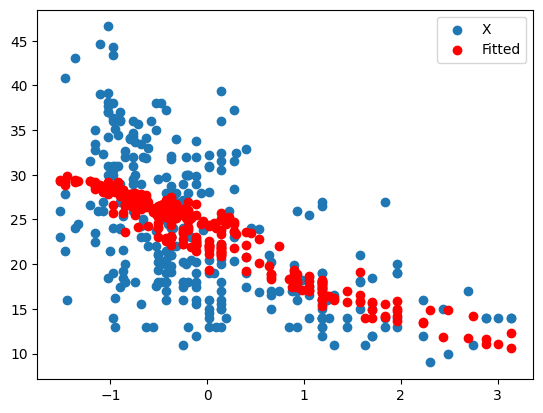In :
model.alpha_

Out:
10.0
In :
pd.DataFrame({"feature": model.feature_names_in_,
"coefficients":model.coef_})

Out:
feature coefficients
0 displacement -2.074038
1 horsepower -1.827595
2 weight -1.040466

### ElasticNet¶

Finally, ElasticNet combines both Lasso and Ridge penalties so you can get the best of both worlds:

In :
model = ElasticNetCV(cv=5).fit(X_train, y_train)

print(model.score(X_test, y_test))
y_fitted = model.predict(X_train)
plt.scatter(X_train[["horsepower"]], y_train, label="X")
plt.scatter(X_train[["horsepower"]], y_fitted, label="Fitted", c="r")
plt.legend()
plt.show()

0.27858321488177085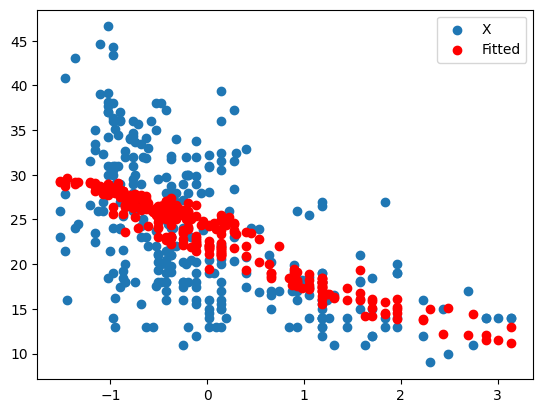We can get the l1_ratio_ back out from here too. The L1 ratio in this case describes the relative weight it gives the Lasso vs Ridge parameters.

In :
model.l1_ratio_

Out:
0.5
In :
model.alpha_

Out:
0.14043693611641683
In :
pd.DataFrame({"feature": model.feature_names_in_,
"coefficients":model.coef_})

Out:
feature coefficients
0 displacement -1.909155
1 horsepower -1.753583
2 weight -1.137835

# Interim Summary¶

So hopefully this helps you understand how to implement Lasso, Ridge, and ElasticNet in python using sklearn. These models would be my go-to to try to estimate feature importance and feature selection because they retain much of the interpretability of Linear Regression.

But, what about an even more flexible model that could fit non-linear relationships in your data? At this point we must pursue more flexible but less interpretable models like popular tree-based models (Random Forest, XGBoost, etc) or less-popular approaches like Support Vector Regression (SVR).

## What is Support Vector Regression?¶

In Support Vector Regression (SVR), the separating hyperplane from Support Vector Machines (SVM) is instead used as the regression line and the decision boundary is used to determine best fit. The benefit of using SVR is that it can capture non-linearity and is robust to outliers and can offer a very flexible fit (with all of those downsides). So in a prediction/forecasting setting this might be very accurate, but you don't get interpretability or inference.

## Example: Support Vector Regression in sklearn¶

For this first example, we'll re-do our initial Linear Regression example of modeling mpg with displacement and plot the fitted data:

In :
from sklearn.svm import SVR

In :
x = X[["displacement"]]

model = SVR(C=1.0, epsilon=0.2, kernel='rbf')
model.fit(x, y)
R_2 = model.score(x, y)

# print the score rounded to two digits
print("R^2 Value: ", np.round(R_2,2))

y_fitted = model.predict(x)
plt.scatter(x, y, label="Actuals")
plt.scatter(x, y_fitted, label="Fitted Values", c="r")
plt.xlabel("Displacement")
plt.ylabel("mpg")
plt.title("SVR with C=1")
plt.legend()
plt.show()

R^2 Value:  0.68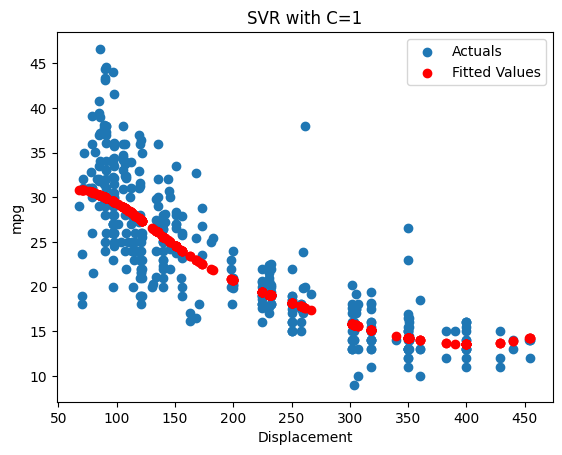Note that we now have a curved fit in this example, even with the default $C$ value of 1.0. In SVR, $C$ represents the inverse of the regularization parameter that we had seen before. So, the lower the value of $C$, the higher the strength of regularization. Another way to think about it is with low $C$ you get a smoother curve and with high $C$ you can get a more 'wiggly' curve.

In :
model = SVR(C=100.0, epsilon=0.2, kernel='rbf')
model.fit(x, y)
R_2 = model.score(x, y)

# print the score rounded to two digits
print("R^2 Value: ", np.round(R_2,2))

y_fitted = model.predict(x)
plt.scatter(x, y, label="Actuals")
plt.scatter(x, y_fitted, label="Fitted Values", c="r")
plt.xlabel("Displacement")
plt.ylabel("mpg")
plt.title("SVR with C=100")
plt.legend()
plt.show()

R^2 Value:  0.69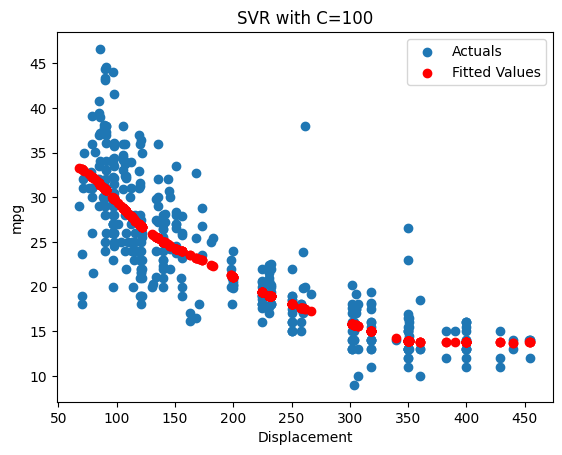So you might be thinking "that's a great $R^2$ value!", but we had just fit the model on the entire dataset. In practice we'll need to use our training data to fit the model and then evaluate the model performance on the 'unseen' test data:

In :
x_train = X_train[["displacement"]]
x_test = X_test[["displacement"]]

model = SVR(C=100.0, epsilon=0.2, kernel='rbf')
model.fit(x_train, y_train)
R_2 = model.score(x_test, y_test)

# print the score rounded to two digits
print("R^2 Value: ", np.round(R_2,2))

y_fitted = model.predict(x_train)
plt.scatter(x_train, y_train, label="Actuals")
plt.scatter(x_train, y_fitted, label="Fitted", c="r")
plt.title("SVR with C=100")
plt.xlabel("scaled displacement")
plt.ylabel("mpg")
plt.legend()
plt.show()

R^2 Value:  0.11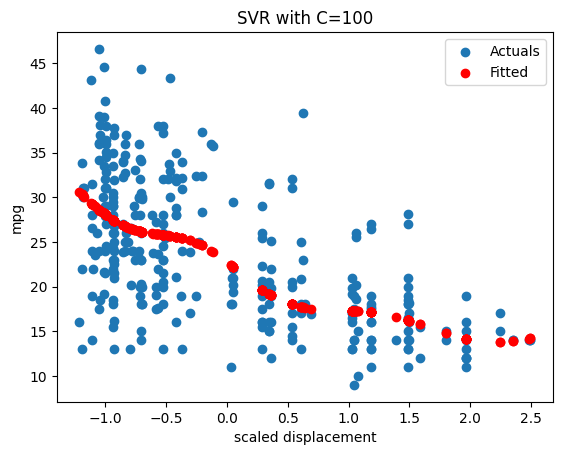And we see that the fit looks pretty good, but what's happening with the test set to get such a low $R^2$?

In :
# print the score rounded to two digits
y_pred = model.predict(x_test)

plt.scatter(x_test, y_test, label="Actuals")
plt.scatter(x_test, y_pred, label="Fitted", c="r")
plt.title("SVR with C=100")
plt.xlabel("scaled displacement")
plt.ylabel("mpg")
plt.legend()
plt.show()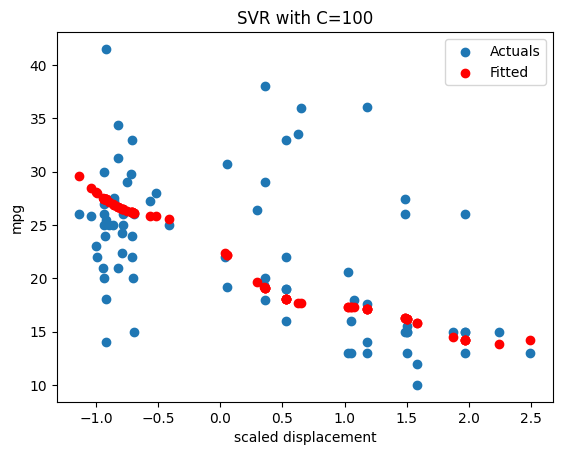And here we may have a case of overfitting where we set $C$ too high.

Instead, we could try a less flexible model and then we would hope to improve the model performance on the test set. Next, we'll set the C back down to 1 and use a linear kernel instead of rbf:

In :
x_train = X_train[["displacement"]]
x_test = X_test[["displacement"]]

model = SVR(C=1, epsilon=0.2, kernel='linear')
model.fit(x_train, y_train)
R_2 = model.score(x_test, y_test)

# print the score rounded to two digits
print("R^2 Value: ", np.round(R_2,2))

y_fitted = model.predict(x_train)
plt.scatter(x_train, y_train, label="Actuals")
plt.scatter(x_train, y_fitted, label="Fitted", c="r")
plt.title("SVR with C=1 and linear kernel")
plt.xlabel("scaled displacement")
plt.ylabel("mpg")
plt.legend()
plt.show()

R^2 Value:  0.2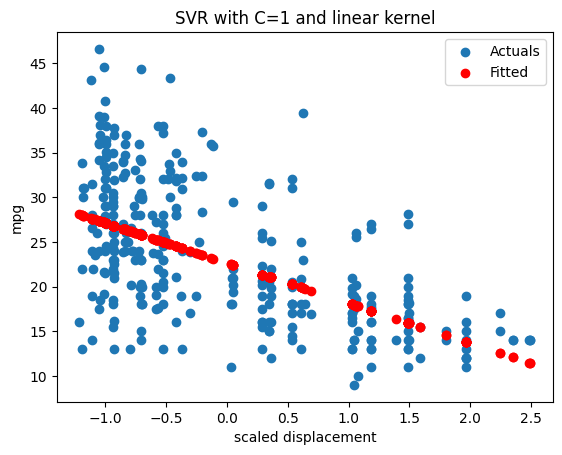And we notice that this starts looking a little more like linear regression in that it did a linear fit. You can even get the coefficients and intercept back out:

In :
print("model coefficient(s):", model.coef_)
print("model intercept:", model.intercept_)

model coefficient(s): [[-4.49507286]]
model intercept: [22.64249525]


### SVR with all predictors¶

Now we set the regularization strength back down to 1.0 and fit on all of the predictors:

In :
model = SVR(C=1.0, epsilon=0.2, kernel='rbf')
model.fit(X_train, y_train)
R_2 = model.score(X_test, y_test)

# print the score rounded to two digits
print("R^2 Value: ", np.round(R_2,2))

y_fitted = model.predict(X_train)
plt.scatter(x_train, y_train, label="Actuals")
plt.scatter(x_train, y_fitted, label="Fitted", c="r")
plt.xlabel("scaled displacement")
plt.ylabel("mpg")
plt.legend()
plt.title("SVR with all predictors, C=1")
plt.show()

R^2 Value:  0.21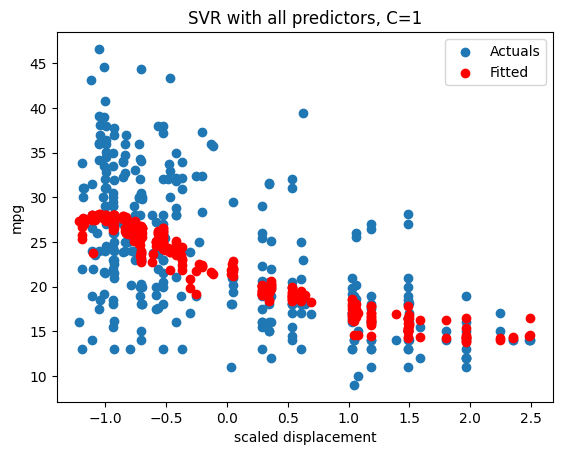With SVR we can't get regression coefficients out unless we use a linear kernel. The documentation suggests that we use LinearSVR instead of calling SVR(kernel='linear') because it offers more flexibility and it's more performant:

LinearSVR

In :
from sklearn.svm import LinearSVR

In :
model = LinearSVR(C=1.0, epsilon=0.2)
model.fit(X_train, y_train)
R_2 = model.score(X_test, y_test)

# print the score rounded to two digits
print("R^2 Value: ", np.round(R_2,2))

y_fitted = model.predict(X_train)
plt.scatter(x_train, y_train, label="Actuals")
plt.scatter(x_train, y_fitted, label="Fitted", c="r")
plt.xlabel("scaled displacement")
plt.ylabel("mpg")
plt.legend()
plt.title("LinearSVR with all predictors, C=1")
plt.show()

R^2 Value:  0.2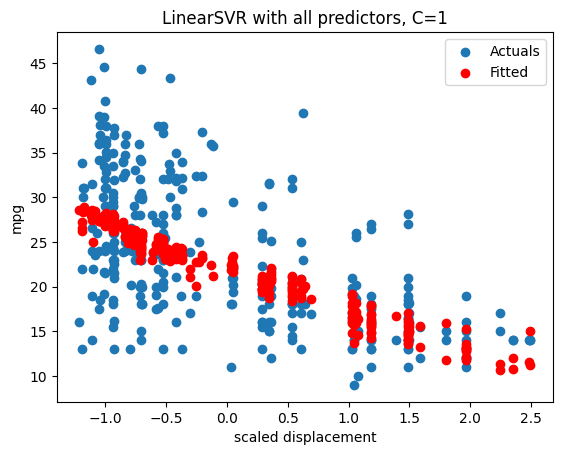In :
pd.DataFrame({"feature": model.feature_names_in_,
"coefficients":model.coef_})

Out:
feature coefficients
0 displacement -2.024878
1 horsepower -0.551462
2 weight -2.430801

## SVR Summary¶

So you can use SVR as a more flexible regression option with a regularization penalty (like we have with Lasso/Ridge/ElasticNet) with the same caveats around the bias-variance tradeoff. Another benefit of this model is that it can handle non-linearity and is robust to outliers. There are computational issues with large datasets but in the kind of excel-friendly datasets that we see in FP&A this wouldn't be that big of a problem.

So you might get a good predictive model from this where you want flexibility and the way to cover non-linear relationships. However, we lose a lot in terms of interpretability with this kind of model compared to Lasso and Ridge, and we no longer get the feature selection ability that we had before.

In [ ]:


In [ ]: